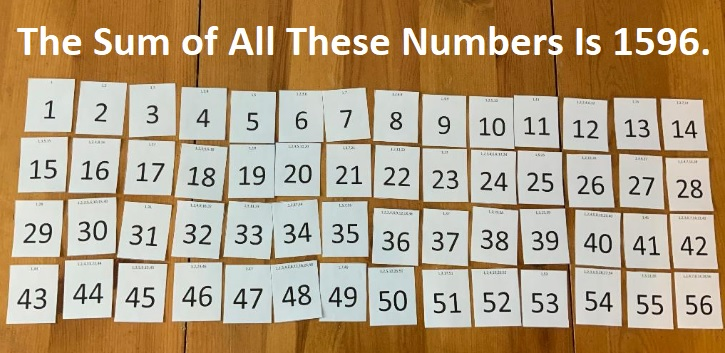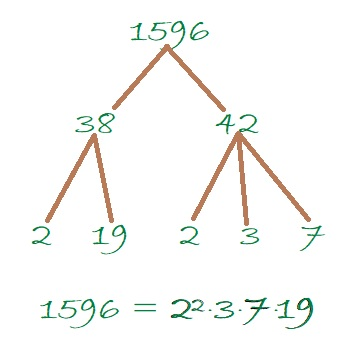# How Much of My \$1596 Will the Taxman Get?

Contents

### Today’s Puzzle is a Game Called Taxman:

Tax season in the United States began just a few days ago. Figuring taxes is no fun, but playing Taxman is.

You see 56 cards below. Imagine that they are 56 envelopes, each containing the dollar amount written on them.You can take whatever envelope you’d like, but the taxman must be able to take at least one envelope when you do. What envelopes will the taxman take when it is his turn? He will take EVERY available envelope that has a number on it that is a factor of the envelope you took. So if you started off taking envelope 56, the taxman would take envelopes 1, 2, 4, 7, 8, 14, and 28. Thus the taxman would start off getting a whole lot more money than you while also limiting envelopes you can choose later. For example, you wouldn’t be able to pick any prime numbers after taking 56  because the 1 would be gone, and the taxman must be able to take one envelope with every choice you make. When you get to the point that there are no more envelopes that you can take, the taxman gets to take ALL the remaining envelopes. You want the taxman to get less money than you do, and hopefully as little money as possible. I’ve included the factors of each of the numbers at the top of each “envelope” to make the game easier for both you and the taxman. You can print “envelopes” from this excel file Taxman & 1537-1544.  You can have a friend be the taxman as you play or you can play both roles.

### How I Played the Game:

I took envelopes as I asked myself these questions:

What is the largest prime number on the table? 53. I take 53, and the only envelope the taxman gets is 1.

What is the largest number that is a prime number squared? 49. I take 49, and the only envelope left for the taxman to take is 7.

What is the largest multiple of 7 that has only one other available factor? 35. I take 35, and the only envelope the taxman can still get is 5.

What is the largest multiple of 5 that has only one other available factor? and so forth.

Here is the order I took the envelopes. It is not the only possible order to use, but it was one in which there were only nine cards at the end of the game for the taxman to claim.The winner of the game is the one with the most money at the end of the game.

### How Do We Know Who Won the Game?

We could add up all the money I got and compare it with all the money the taxman got, but that wouldn’t be much fun. We could add up all the taxman money and subtract it from 1596 to find my total, but I prefer a different way: I remove all the taxman’s money from the table and try to mix envelopes to match mine. I start with my envelope with the most cash, 56 = 8 + 1 + 47, so I put the taxman’s envelopes with those numbers next to my 56.

Matching the “envelopes” up like this will take some time, but it feels like playing a game. It may have taken me as long to play this part of the game as it took me to play Taxman because sometimes I had to remove perfectly good sums in order to use all the taxman’s envelopes in a sum. Here is how I matched the envelopes:I know that I won the game because I had at least one envelope without a sum next to it. I can determine by  how much I won the game by adding 35 + 33 + 46 + 39 + 27 + 38 + 20 + 30 + 42. That sum tells me I won the game by \$310.

How much did the taxman get?
(1596-310)/2 = 643.

How much did I get to keep?
643 + 310 = 953.

### A Factor Tree for 1596:

If you know that 40² = 1600, and 1600 – 4 = 1596, we might recognize that 1596 is the difference of two perfect squares: 40² – 2². Then we can also know that
1596 = (40 – 2)(40 + 2) = 38 × 42.

Let’s make a factor tree from the factor pair 38 × 42:Since 1596 has several factors, it has several other possible factor trees.

### Factors of 1596:

• 1596 is a composite number.
• Prime factorization: 1596 = 2 × 2 × 3 × 7 × 19, which can be written 1596 = 2² × 3 × 7 × 19.
• 1596 has at least one exponent greater than 1 in its prime factorization so √1596 can be simplified. Taking the factor pair from the factor pair table below with the largest square number factor, we get √1596 = (√4)(√399) = 2√399
• The exponents in the prime factorization are 2, 1, 1, and 1. Adding one to each exponent and multiplying we get (2 + 1)(1 + 1)(1 + 1)(1 + 1) = 3 × 2 × 2 × 2 = 24. Therefore 1596 has exactly 24 factors.
• The factors of 1596 are outlined with their factor pair partners in the graphic below.### More about the Number 1596:

The reason that I was dividing up \$1596 between me and the taxman is that 1596 is the 56th triangular number. It is the 56th triangular number because
(56)(57)/2 = 1596.

1596 is the difference of two squares in four different ways:

1596 is the sum of the first 15 Fibonacci numbers, which also means it is one number less than the 17th Fibonacci number.This site uses Akismet to reduce spam. Learn how your comment data is processed.